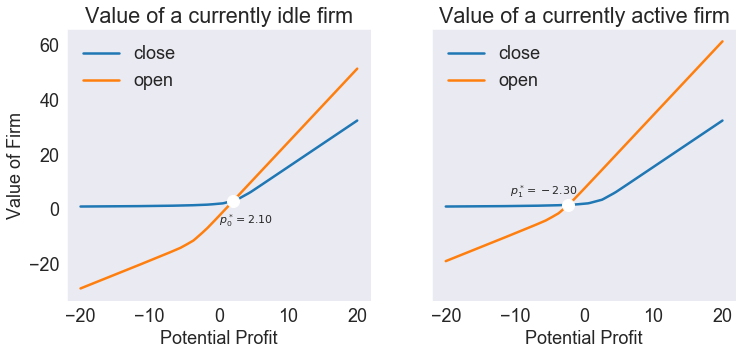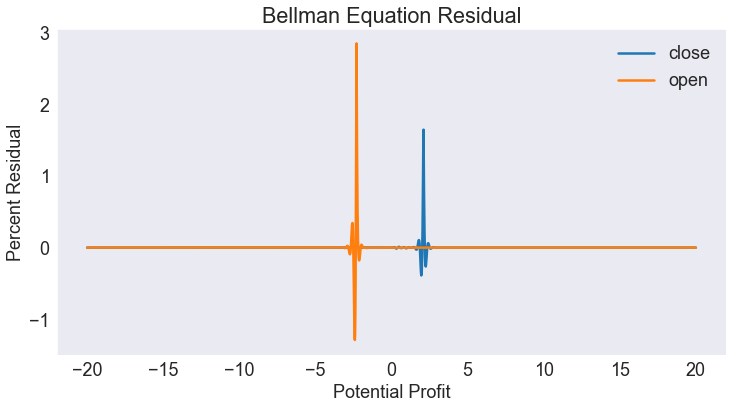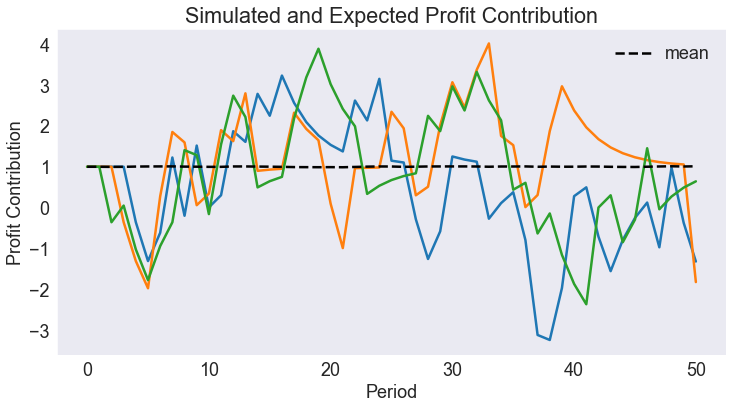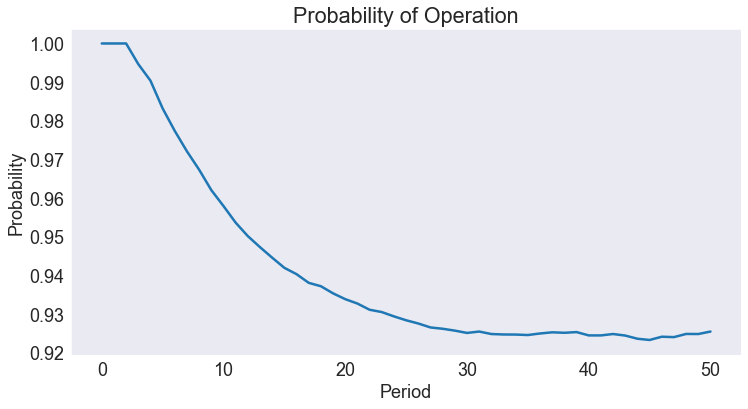# Industry Entry-Exit Model¶

A firm operates in an uncertain profit environment. At the beginning of each period, the firm observes its potential short-run operating profit over the coming period $\pi$, which may be negative, and decides whether to operate, making a short-run profit $\pi$, or not operate, making a short-run profit $0$. Although the firm faces no fixed costs, it incurs a shutdown cost $K_0$ when it closes and a start-up cost $K_1$ when it reopens. The short-run profit $\pi$ is an exogenous continuous-valued Markov process \begin{equation} \pi_{t+1} = h(\pi_t, \epsilon_{t+1}) \end{equation}

What is the optimal entry-exit policy? In particular, how low must the short-run profit be for an operating firm to close, and how high must the short-run profit be for nonoperating firm to reopen?

This is an infinite horizon, stochastic model with time $t$ measured in years. The state variables \begin{align} \pi &\in (−\infty,\infty)\\ d &\in \{0, 1\} \end{align}

are the current short-run profit, a continuous variable, and the operational status of the firm, a binary variable that equals 1 if the firm is operating and 0 if the firm is not operating. The choice variable \begin{equation} j \in \{0, 1\} \end{equation}

is the operating decision for the coming year, a binary variable that equals 1 if the firm operates and 0 if does not operate. The state transition function is \begin{equation} g(\pi, d, j, \epsilon) = h(\pi, \epsilon), j) \end{equation}

The reward function is \begin{equation} f(\pi, d, j) = \pi j − K_1(1 − d)j − K_0 d(1 − j) \end{equation}

The value of the firm, given that the current short-run profit is $\pi$ and the firm's operational status is $d$, satisfies the Bellman equation \begin{equation} V(\pi,d) = \max_{j=0,1}\left\{\pi j − K_1(1−d)j − K_0d(1−j) + \delta E_\epsilon V\left(h(\pi,\epsilon),j\right)\right\} \end{equation}

In :
import numpy as np
import pandas as pd
import matplotlib.pyplot as plt
from compecon import BasisSpline, DPmodel,qnwnorm, demo


### The reward function¶

The reward function is \begin{equation} f(\pi, d, j) = \pi j − K_1(1 − d)j − K_0 d(1 − j) \end{equation}

where the exit cost is $K_0=0$ and the entry cost is $K_1=10$.

In :
K0     = 0.0
K1     = 10

def profit(p, x, d, j):
return p * j - K1 * (1 - d) * j - K0 * d * (1 - j)


### The transition function¶

Assuming that the short-run profit $\pi$ is an exogenous Markov process \begin{equation} \pi_{t+1} = g(\pi_t,\epsilon_{t+1}) = \bar{\pi} + \gamma(\pi_t − \bar{\pi}) + \epsilon_{t+1} \end{equation}
where $\bar{\pi}=1.0$ and $\gamma=0.7$.

In :
pbar   = 1.0
gamma  = 0.7

def transition(p, x, d, j, in_, e):
return pbar + gamma * (p - pbar) + e


In the transition function $\epsilon_t$ is an i.i.d. normal(0, $σ^2$), with $\sigma=1$. We discretize this distribution by using a discrete distribution, matching the first 10 moments of the normal distribution.

In :
m = 5  # number of profit shocks
sigma  = 1.0
[e,w] = qnwnorm(m,0,sigma **2)


The collocation method calls for the analyst to select $n$ basis functions $\varphi_j$ and $n$ collocation nodes $(\pi_i,d_i)$, and form the value function approximant $V(\pi,d) \approx \sum_{j=1}^{n} c_j\varphi_j(\pi,d)$ whose coefficients $c_j$ solve the collocation equation

\begin{equation} \sum_{j=1}^{n} c_j\varphi_j(\pi_i,d_i) = \max_{x\in\{0,1\}}\left\{\pi_i x − K_1(1−d_i)x − K_0 d_i(1−x) + \delta\sum_{k=1}^{m}\sum_{j=1}^{n}w_k c_j \varphi_j(\hat{\pi}_{ik},x)\right\} \end{equation}

where $\hat\pi_{ik}=g(\pi_i,\epsilon_k)$ and where $\epsilon_k$ and $w_k$ represent quadrature nodes and weights for the normal shock.

For the approximation, we use a cubic spline basis with $n=250$ nodes between $p_{\min}=-20$ and $p_\max=20$.

In :
n = 250
pmin = -20
pmax =  20
basis = BasisSpline(n, pmin, pmax, labels=['profit'])
print(basis)

A 1-dimension Cubic spline basis:  using 250 Canonical nodes and 250 polynomials
___________________________________________________________________________
profit: 250 nodes in [-20.00,  20.00]

===========================================================================
WARNING! Class Basis is still work in progress


Discrete states and discrete actions are

In :
dstates = ['idle', 'active']
dactions = ['close', 'open']


The Bellman equation is represeted by a DPmodel object, where we assume a discount factor of $\delta=0.9$. Notice that the discrete state transition is deterministic, with transition matrix \begin{equation} h=\begin{bmatrix}0&0\\1&1\end{bmatrix} \end{equation}

In :
model = DPmodel(basis,
profit, transition,
i=dstates,
j=dactions,
discount=0.9, e=e, w=w,
h=[[0, 0], [1, 1]])


## SOLUTION¶

To solve the model, we simply call the solve method on the model object.

In :
S = model.solve(show=True)

Solving infinite-horizon model collocation equation by Newton's method
iter change       time
------------------------------
0       6.0e+01    0.0798
1       3.2e+01    0.1478
2       2.9e+00    0.2258
3       4.3e-01    0.2918
4       4.4e-14    0.3597
Elapsed Time =    0.36 Seconds

Out:
profit i value resid j* value[close] value[open]
profit
idle -20.000000 -20.000000 0 0.894342 0.000000e+00 close 0.894342 -29.105658
-19.983994 -19.983994 0 0.894542 -5.251344e-11 close 0.894542 -29.089452
-19.967987 -19.967987 0 0.894743 -5.991030e-11 close 0.894743 -29.073245
-19.951981 -19.951981 0 0.894943 -2.191847e-11 close 0.894943 -29.057038
-19.935974 -19.935974 0 0.895144 4.235901e-11 close 0.895144 -29.040830

### Plot Action-Contingent Value Functions¶

In :
fig1,axs = plt.subplots(1,2,sharey=True, figsize=[12,5])
offs = [(4, -8), (-8, 5)]
msgs = ['Profit Entry', 'Profit Exit']

for i,iname in enumerate(dstates):
plt.axes(axs[i])
subdata = S.loc[iname,['value[close]', 'value[open]']]
plt.plot(subdata)
plt.title('Value of a currently ' + iname + ' firm')
plt.xlabel('Potential Profit')
if not i: plt.ylabel('Value of Firm')
plt.legend(dactions)

subdata['v.diff'] = subdata['value[open]'] - subdata['value[close]']
pcrit = np.interp(0, subdata['v.diff'], subdata.index)
vcrit  = np.interp(pcrit, subdata.index, subdata['value[close]'])
demo.annotate(pcrit, vcrit, f'$p^*_{i:d} = {pcrit:.2f}$', 'wo', offs[i], fs=11, ms=12,)
print(f'{msgs[i]:12s} = {pcrit:5.2f}')


Profit Entry =  2.10
Profit Exit  = -2.30### Plot Residual¶

We normalize the residuals as percentage of the value function. Notice the spikes at the "Profit entry" and "Profit exit" points.

In :
S['resid2'] = 100 * (S.resid / S.value)
fig2 = demo.figure('Bellman Equation Residual','Potential Profit','Percent Residual')
demo.qplot('profit','resid2','i',S)
plt.legend(dactions)

Out:
<matplotlib.legend.Legend at 0x283ba68d608>## SIMULATION¶

We simulate the model 50000 times for a time horizon $T=50$, starting with an operating firm ($d=1$) at the long-term profit mean $\bar{\pi}$. To be able to reproduce these results, we set the random seed at an arbitrary value of 945.

In :
T = 50
nrep = 50000
p0 = np.tile(pbar, (1, nrep))
d0 = 1
data = model.simulate(T, p0, d0, seed=945)

In :
f = '\t{:21s} = {:5.2f}'
print('\nErgodic Means')
print(f.format('Profit Contribution', data['profit'].mean()))
print(f.format('Activity', (data['i'] == 'active').mean()))
print('\nErgodic Standard Deviations\n')
print(f.format('Profit Contribution', data['profit'].std()))
print(f.format('Activity', (data['i'] == 'active').std()))

Ergodic Means
Profit Contribution   =  1.00
Activity              =  0.94

Ergodic Standard Deviations

Profit Contribution   =  1.37
Activity              =  0.24

In :
ergodic = pd.DataFrame({
'Ergodic Means' : [data['profit'].mean(), (data['i'] == 'active').mean()],
'Ergodic Standard Deviations': [data['profit'].std(), (data['i'] == 'active').std()]},
index=['Profit Contribution', 'Activity'])

ergodic.round(2)

Out:
Ergodic Means Ergodic Standard Deviations
Profit Contribution 1.00 1.37
Activity 0.94 0.24

### Plot Simulated and Expected Continuous State Path¶

In :
subdata = data[data['_rep'].isin(range(3))]

fig3 = demo.figure('Simulated and Expected Profit Contribution', 'Period','Profit Contribution')
demo.qplot('time','profit','_rep',subdata)
plt.plot(data[['time','profit']].groupby('time').mean(),'k--',label='mean')
plt.legend()

Out:
<matplotlib.legend.Legend at 0x283ba876488>### Plot Expected Discrete State Path¶

In :
fig4 = demo.figure('Probability of Operation', 'Period', 'Probability')
data['ii'] = data['i'] == 'active'
plt.plot(data[['time','ii']].groupby('time').mean())

Out:
[<matplotlib.lines.Line2D at 0x283ba8c5948>]In :
#demo.savefig([fig1,fig2,fig3,fig4])